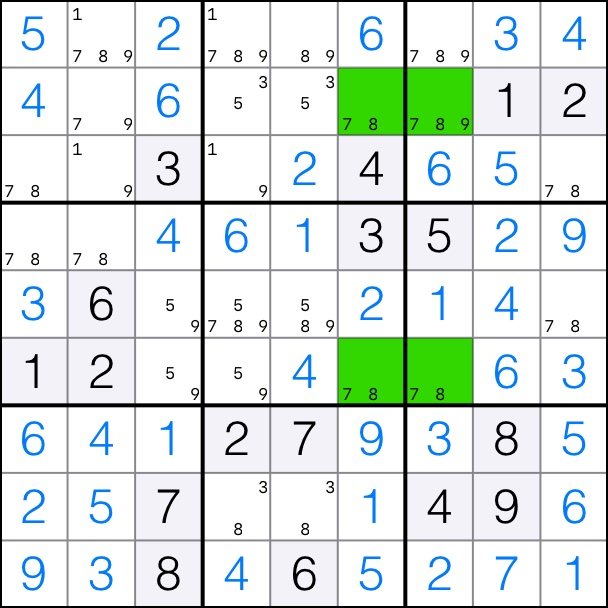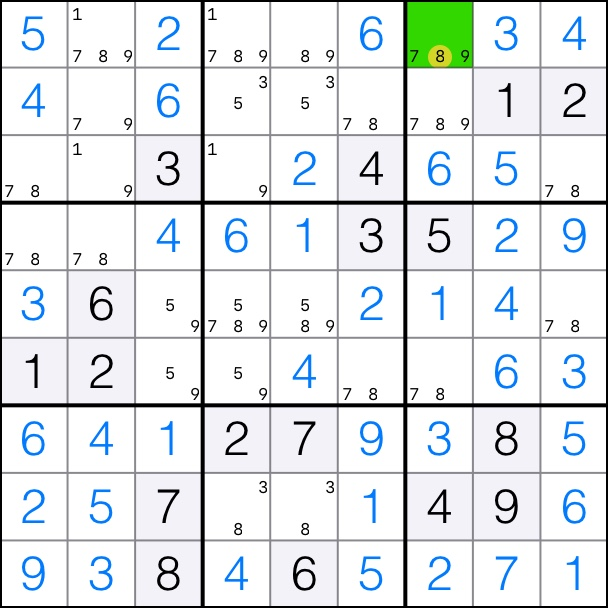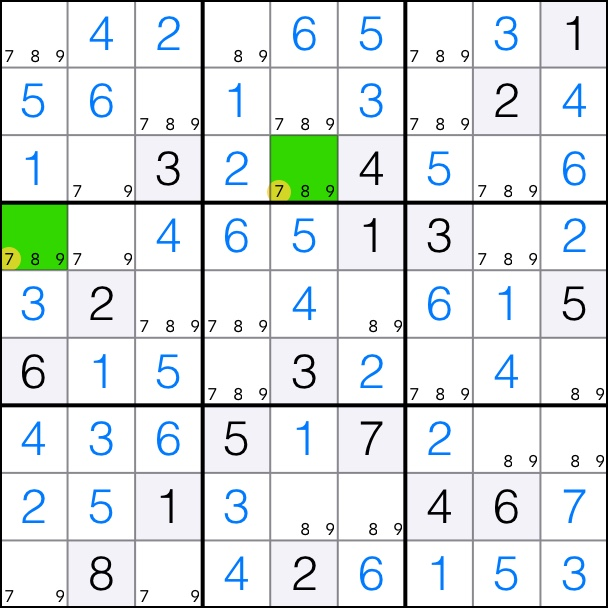## Description

The X-Wing is a rectangle of 4 cells which all contain a given candidate. In a given set of 2 rows, the candidate must appear only twice and in the exact same 2 columns. Similarly, in a given set of 2 columns, the candidate must appear only twice and in the exact same 2 rows.

## Reasoning

Because the candidate appears exactly 2 times in each row and there are 2 rows, it means that the candidate must appear exactly twice in these 4 cells. Therefore it cannot apear anywhere else in the 2 columns. Similarly for the column based X-Wing.

## Examples

### Row Example7 forms an X-Wing on rows 2 and 6.That means that candidates 7 can be removed from all other cells in columns 6 and 7, cell [1,7].

### Column Example7 forms an X-Wing on columns 2 and 8.That means that candidates 7 can be removed from all other cells in rows 3 and 4, cells [3,5] and [4,1].

## Algorithm

1. Fill in all the pencil marks in the puzzle.
2. For every available digit 1 to 9
1. For every combination of 2 rows
1. Find the if digit candidate is in the same exact 2 columns for both rows. The digit cannot be in any other columns.
2. If yes, then we can remove all the candidates from those columns that are not in our 2 rows.
2. For every combination of 2 columns
1. Find the if digit candidate is in the same exact 2 rows for both columns. The digit cannot be in any other row.
2. If yes, then we can remove all the candidates from those rows that are not in our 2 columns.

## Practice Puzzles

If you tap on the following links on an iOS device which has the Sudoku Tutor app installed, it will launch the app and open the practice puzzle. Tap hint once the puzzle is open to see the strategy in action.

Sample Puzzle 1
Sample Puzzle 2
Sample Puzzle 3

## Next Step

Back to Sudoku Solvers or continue to next algorithm XY-Wing